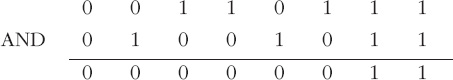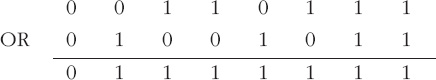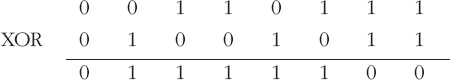Representing and manipulating data in computers

Start this free course now. Just create an account and sign in. Enrol and complete the course for a free statement of participation or digital badge if available.

Free course

# 8.6 Summary

The logic operations introduced here are summarised in Table 1, which is an example of what is known as a ‘truth table’. It shows what the result (‘output’) of each logic operation is for all possible combinations of ‘input’ values. You may find this format a useful one for remembering the various logic operations.

## Table 1: Summary of the logic operations NOT, AND, OR and XOR

 Operation Inputs Output NOT 0 — 1 1 — 0 AND 0 0 0 0 1 0 1 0 0 1 1 1 OR 0 0 0 0 1 1 1 0 1 1 1 1 XOR 0 0 0 0 1 1 1 0 1 1 1 0

## Activity 28 (Self assessment)

1. If A = 0011 0111 and B = 0100 1011, find

1. NOT A

2. A AND B

3. A OR B

4. A XOR B

2.

1. Is A AND B=B AND A?

2. Is A OR B=B OR A?

3. Is A XOR B=B XOR A?

1.

1. NOT A is the complement of A, which is 1100 1000.So A AND B is 0000 0011.

2.So A OR B is 0111 1111.

3.So A XOR B is 0111 1100.

2.

1. Yes

2. Yes

3. Yes